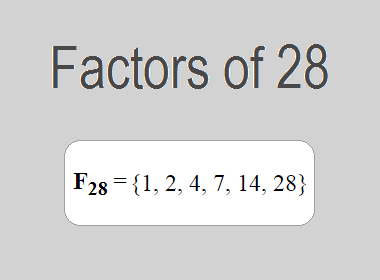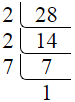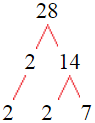# Factors of 28The factors of 28 are 1, 2, 4, 7, 14, and 28 i.e. F28 = {1, 2, 4, 7, 14, 28}. The factors of 28 are all the numbers that can divide 28 without leaving a remainder.

We can check if these numbers are factors of 28 by dividing 28 by each of them. If the result is a whole number, then the number is a factor of 28. Let's do this for each of the numbers listed above:

·        1 is a factor of 28 because 28 divided by 1 is 28.

·        2 is a factor of 28 because 28 divided by 2 is 14.

·        4 is a factor of 28 because 28 divided by 4 is 7.

·        7 is a factor of 28 because 28 divided by 7 is 4.

·        14 is a factor of 28 because 28 divided by 14 is 2.

·        28 is a factor of 28 because 28 divided by 28 is 1.

## How to Find Factors of 28?

1 and the number itself are the factors of every number. So, 1 and 28 are two factors of 28. To find the other factors of 28, we can start by dividing 28 by the numbers between 1 and 28. If we divide 28 by 2, we get a remainder of 0. Therefore, 2 is a factor of 28. If we divide 28 by 3, we get a remainder of 1. Therefore, 3 is not a factor of 28.

Next, we can check if 4 is a factor of 28. If we divide 28 by 4, we get a remainder of 0. Therefore, 4 is a factor of 28. We can continue this process for all the possible factors of 28.

Through this process, we can find that the factors of 28 are 1, 2, 4, 7, 14, and 28. These are the only numbers that can divide 28 without leaving a remainder.

********************

********************

## Properties of the Factors of 28

The factors of 28 have some interesting properties. One of the properties is that the sum of the factors of 28 is equal to 56. We can see this by adding all the factors of 28 together:

1 + 2 + 4 + 7 + 14 + 28 = 56

Another property of the factors of 28 is that the prime factors of 28 are 2, and 7 only.

## Applications of the Factors of 28

The factors of 28 have several applications in mathematics. One of the applications is in finding the highest common factor (HCF) of two or more numbers. The HCF is the largest factor that two or more numbers have in common. For example, to find the HCF of 28 and 35, we need to find the factors of both numbers and identify the largest factor they have in common. The factors of 28 are 1, 2, 4, 7, 14, and 28. The factors of 35 are 1, 5, 7, and 35. The largest factor that they have in common is 7. Therefore, the HCF of 28 and 35 is 7.

Another application of the factors of 28 is in prime factorization. Prime factorization is the process of expressing a number as the product of its prime factors. The prime factors of 28 are 2, and 7 since these are the only prime numbers that can divide 28 without leaving a remainder. Therefore, we can express 28 as:

28 = 2 × 2 × 7

We can do prime factorization by division and factor tree method also. Here is the prime factorization of 28 by division method,28 = 2 × 2 × 7

Here is the prime factorization of 28 by the factor tree method,28 = 2 × 2 × 7

## Conclusion

The factors of 28 are the numbers that can divide 28 without leaving a remainder. The factors of 28 are 1, 2, 4, 7, 14, and 28. The factors of 28 have some interesting properties, such as having a sum of 56. The factors of 28 have several applications in mathematics, such as finding the highest common factor and prime factorization.